# Triangles

Here we will learn about triangles, including what a triangle is and how to solve problems involving their sides and their angles.

There are also triangles worksheets based on Edexcel, AQA and OCR exam questions, along with further guidance on where to go next if you’re still stuck.

## What are triangles?

Triangles are 2D shapes with three straight sides and three vertices. These are the only two key properties of triangles.

To solve problems involving triangles, we need to be able to gather information about its side lengths and/or its interior angles and apply these to the problem.

The sum of interior angles of a triangle is \bf{180°}.

For example,

29^{\circ}+68^{\circ}+83^{\circ}=180^{\circ}.

If a triangle’s angles are all acute angles (less than 90^{\circ} ), it is sometimes known as an acute triangle.

If a given triangle has one obtuse angle it is sometimes known as an obtuse triangle.

Step-by-step guide: Angles in a triangle

### What are triangles?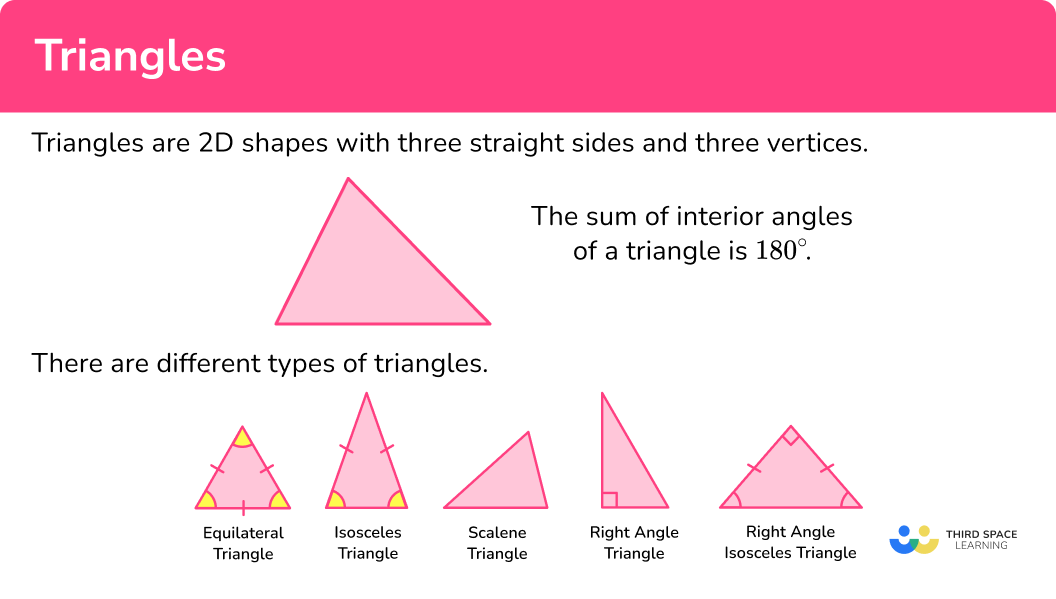### Types of triangles

There are different types of triangles that have different properties.

Being able to recognise different types of triangles is known as classifying triangles.

Step-by-step guide: Types of triangles

### Equilateral triangles

An equilateral triangle has the following properties,

• All sides are equal length.
• All angles are equal size (60^{\circ}) .

An equilateral triangle has 3 lines of symmetry. The lines go from one vertex to the middle of the opposite side.

The lines of symmetry are angle bisectors of the vertices and are also perpendicular bisectors of the sides of the equilateral triangle.

Step-by-step guide: Equilateral triangles

### Isosceles triangles

An isosceles triangle has the following properties,

• Two sides are equal length.
• Two equal angles.

The two equal angles are called base angles and they are opposite the sides of equal length. The unequal side is known as the base and is opposite the unequal angle.

Step-by-step guide: Isosceles triangles

### Scalene triangles

A scalene triangle has the following properties,

• No equal sides.
• No equal angles.

Step-by-step guide: Scalene triangles

### Right-angled triangles

A right-angled triangle has the following property,

• One right angle.

The longest side of a right-angled triangle is opposite the right-angle. It is called the hypotenuse.

The Pythagorean theorem is used to determine missing side lengths of a right-angled triangle. If a question involves both the sides of the triangle and one of the angles, we could use trigonometry.

A special case of a right-angled triangle is a right-angled triangle which also has two equal sides. It can be known as an isosceles right triangle or an isosceles right-angled triangle. The two equal angles will both be 45^{\circ}.

Step-by-step guide: Right angle triangle

### Area of a triangle

The area of a triangle can be found by using the formula,

A=\frac{1}{2}bh ,

where b is the base length and h is the perpendicular height of the triangle.

Sometimes these values need to be calculated.

The area of a triangle is given in square units.

Step-by-step guide: Area of a triangle

### Impossible triangles

It is impossible for a triangle to contain more than one obtuse angle as the sum of angles in a triangle is 180^{\circ}.

For example,

• A triangle contains the three angles 98^{\circ}, \ 91^{\circ} and 5^{\circ}.
This gives us the angle sum of 98+91+5=194^{\circ} and so as angles in a triangle always total 180^{\circ}. This is an impossible triangle.

• A triangle contains two right angles, and a third angle x^{\circ}.
The sum of angles in the triangle is therefore 90+90+x=(180+x)^{\circ}.
The value of x must equal 0 for the angles to add to 180^{\circ} and so this is another impossible triangle.

## How to answer questions involving triangles

In order to solve problems involving triangles:

1. Locate known angles and calculate any necessary unknown angles.
2. Locate known sides and calculate any necessary unknown side lengths.
3. Solve the problem using any necessary values.

### Explain how to answer questions involving triangles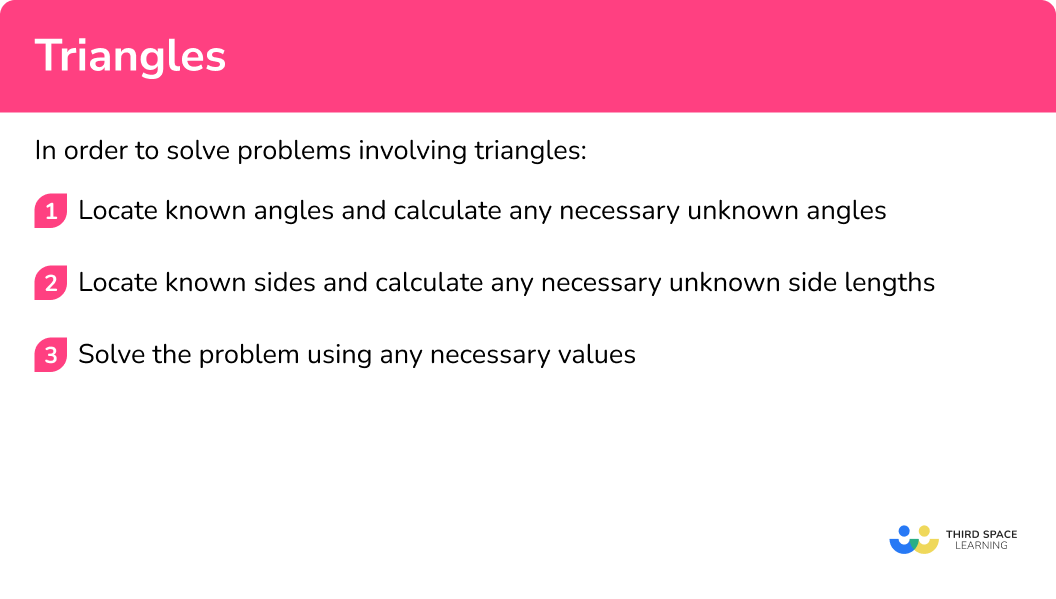## Triangles examples

### Example 1: calculate the missing angle in an isosceles triangle

Find angle x.

1. Locate known angles and calculate any necessary unknown angles.

This problem involves the internal angles of a triangle. The sum of interior angles of a triangle is 180^{\circ}. One angle is given.

As two sides have a dash through them indicating they are the same length, the triangle is isosceles and so the triangle contains the two base angles 63^{\circ} and 63^{\circ}.

2Locate known sides and calculate any necessary unknown side lengths.

As we need to determine the size of angle x and we have information about the other two angles, we can use these to find the missing angle. We do not need to calculate any length of the triangle.

3Solve the problem using any necessary values.

Using the two known angles and the angle fact that the sum of angles in a triangle is 180^{\circ},

180-(2\times 63)=54.

Therefore angle x=54^{\circ}.

### Example 2: finding the missing angle including external angles

Determine the size of angle x.

Locate known angles and calculate any necessary unknown angles.

Locate known sides and calculate any necessary unknown side lengths.

Solve the problem using any necessary values.

### Example 3: finding the missing angle (right-angled isosceles)

Calculate the missing angle x.

Locate known angles and calculate any necessary unknown angles.

Locate known sides and calculate any necessary unknown side lengths.

Solve the problem using any necessary values.

### Example 4: calculating the perimeter

The sides of a triangle are 13 \ cm, 16 \ cm and 21 \ cm. What is the perimeter of the triangle?

Locate known angles and calculate any necessary unknown angles.

Locate known sides and calculate any necessary unknown side lengths.

Solve the problem using any necessary values.

### Example 5: perimeter problem using congruent triangles

Here is a triangle.

Two of these triangles are joined on their shortest sides to make a parallelogram. Calculate the perimeter of the parallelogram.

Locate known angles and calculate any necessary unknown angles.

Locate known sides and calculate any necessary unknown side lengths.

Solve the problem using any necessary values.

### Example 6: perimeter problem using ratio

The perimeter of a triangle is 119 \ cm.

The ratio of the sides of the triangle is 5:4:8.

Determine the length of each side of the triangle.

Locate known angles and calculate any necessary unknown angles.

Locate known sides and calculate any necessary unknown side lengths.

Solve the problem using any necessary values.

### Common misconceptions

• Angles in polygons

Make sure you know your angle properties as getting these confused causes quite a few misconceptions.

◌ Angles in a triangle total 180^{\circ} .

◌ Angles in a quadrilateral total 360^{\circ} .

• Angle facts

Make sure you know your angle properties.

◌ Adjacent angles on a straight line add up to 180^{\circ} .

◌ Vertically opposite angles are equal.

◌ Alternate angles are equal.

◌ Corresponding angles are equal.

### Practice triangle questions

1. Which of these shapes show an equilateral triangle?An equilateral triangle has all equal sides and all equal angles.

2. The sides of a triangle are 4.3 \ cm, 4.7 \ cm, and 43 \ mm. What kind of triangle is it?

ScaleneEquilateralIsoscelesRight-angled43 \ mm is the same as 4.3 \ cm. Since two sides are the same, it is an isosceles triangle.

3. Find angle x.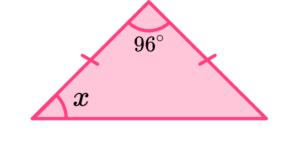84^{\circ}63^{\circ}42^{\circ}96^{\circ}The triangle has two equal side lengths and so it is an isosceles triangle. This means that the base angles will be equal. The interior angles of a triangle sum to 180^{\circ}. Angle x is therefore equal to

(180-96)\div 2=42^{\circ}.

Angle x=42^{\circ}

4. Determine the size of the angle x in the diagram below.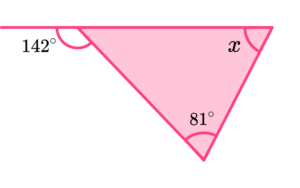142^{\circ}81^{\circ}38^{\circ}61^{\circ}As the sum of adjacent angles is 180^{\circ}, the angle next to 142^{\circ} is equal to 180-142=38^{\circ}.

As the sum of angles in a triangle is 180^{\circ}, the unknown value of x is equal to 180-(81+38)=61^{\circ} and so x=61^{\circ}.

5. Here is a triangle. Two of these triangles are joined on the longest sides to make a quadrilateral. Find its perimeter.12 \ cm24 \ cm19 \ cm14 \ cmThe two congruent triangles will make a rectangle when they are joined on their longest side (the hypotenuse).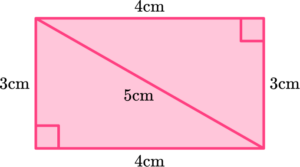The perimeter of the rectangle will be

2\times (3+4)=14.

6. The perimeter of an isosceles triangle is 45 \ cm. The unequal side is half the size of one of the equal sides. Find the length of the unequal side of the isosceles triangle.

9 \ cm15 \ cm18 \ cm12 \ cmThe ratio of the sides of the isosceles triangle would be 1:2:2.The unequal side is the shortest side and can be found by

45\div (1+2+2)=45\div 5=9.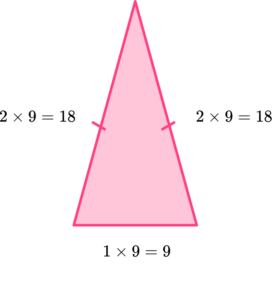The sides of the triangles will be 9 \ cm, 18 \ cm, and 18 \ cm. The shortest side will be 9 \ cm.

### Equilateral triangle GCSE questions

1. Here is a triangle.Draw the line(s) of symmetry of the shape.

(2 marks)For one line of symmetry.

(1)

For three lines of symmetry.

(1)

2. Below is a diagram showing the triangle ABC. The point D lies on the line AC such that BD = CD.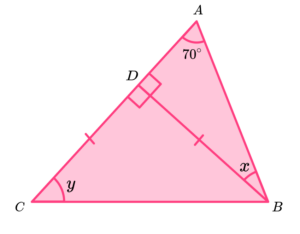(a) Determine the size of angle x.

(b) Find angle y.

(4 marks)

(a)

180-(90+70)

(1)

20^{\circ}

(1)

(b)

(180-90)\div{2}

(1)

45^{\circ}

(1)

3. (a) Write down the coordinates of point B.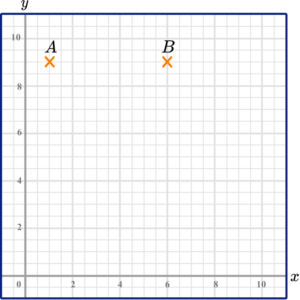(b) Plot the point (1,3) and label it C.

(c) The points A, B and C  make a triangle.

Find the area of the triangle.

(4 marks)

(a) (6,9)

(1)

(b)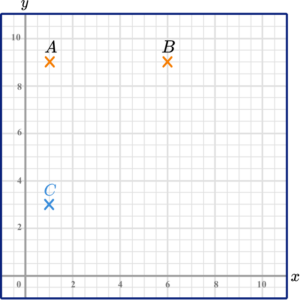(1)

(c)

(6\times 5)\div 2

(1)

15 \ cm^2

(1)

4. The perimeter of a triangle ABC  is 60 \ cm.

The ratio of the sides of the triangle are 2:3:3.
(a) What type of triangle is ABC ?

(b) Find the smallest side of the triangle ABC.

(c) Heron’s formula can be used to find the area of a triangle.

Heron’s formula is

\text{Area}=\sqrt{s(s-a)(s-b)(s-c)}

where s is half the perimeter and a, b and c are the three side lengths.

Use Heron’s formula along with your solution to part b) to find the area of the triangle ABC.

(8 marks)

(a) Isosceles

(1)

(b)

60\div (2+3+3)=60\div 8 \ (=7.5)

(1)

2\times 7.5=15

(1)

(c)

s=60\div 2 (=30)

(1)

The second and third sides are 22.5 \ cm.

(1)

\text{Area}=\sqrt{30(30-15)(30-22.5)(30-22.5)} (=159.0990258…)

(1)

159

(1)

cm^2

(1)

## Learning checklist

You have now learned how to:

• Recognise different types of triangles
• Solve problems involving the angles of a triangle
• Solve problems involving the perimeter of a triangle

## Still stuck?

Prepare your KS4 students for maths GCSEs success with Third Space Learning. Weekly online one to one GCSE maths revision lessons delivered by expert maths tutors.

Find out more about our GCSE maths tuition programme.